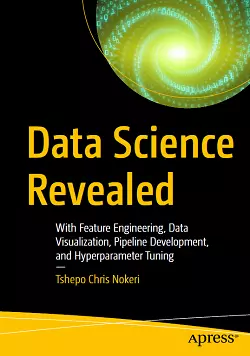# Data Science Revealed### eBook Details:

• Paperback: 272 pages
• Publisher: WOW! eBook (March 24, 2021)
• Language: English
• ISBN-10: 1484268695
• ISBN-13: 978-1484268698

### eBook Description:

Data Science Revealed: With Feature Engineering, Data Visualization, Pipeline Development, and Hyperparameter Tuning

Get insight into data science techniques such as data engineering and visualization, statistical modeling, machine learning, and deep learning. This book teaches you how to select variables, optimize hyper parameters, develop pipelines, and train, test, and validate machine and deep learning models. Each chapter includes a set of examples allowing you to understand the concepts, assumptions, and procedures behind each model.

The Data Science Revealed book covers parametric methods or linear models that combat under- or over-fitting using techniques such as Lasso and Ridge. It includes complex regression analysis with time series smoothing, decomposition, and forecasting. It takes a fresh look at non-parametric models for binary classification (logistic regression analysis) and ensemble methods such as decision trees, support vector machines, and naive Bayes. It covers the most popular non-parametric method for time-event data (the Kaplan-Meier estimator). It also covers ways of solving classification problems using artificial neural networks such as restricted Boltzmann machines, multi-layer perceptrons, and deep belief networks. The book discusses unsupervised learning clustering techniques such as the K-means method, agglomerative and Dbscan approaches, and dimension reduction techniques such as Feature Importance, Principal Component Analysis, and Linear Discriminant Analysis. And it introduces driverless artificial intelligence using H2O.

What You Will Learn

• Design, develop, train, and validate machine learning and deep learning models
• Find optimal hyper parameters for superior model performance
• Improve model performance using techniques such as dimension reduction and regularization
• Extract meaningful insights for decision making using data visualization

After reading this Data Science Revealed: With Feature Engineering, Data Visualization, Pipeline Development, and Hyperparameter Tuning book, you will be able to develop, test, validate, and optimize statistical machine learning and deep learning models, and engineer, visualize, and interpret sets of data.